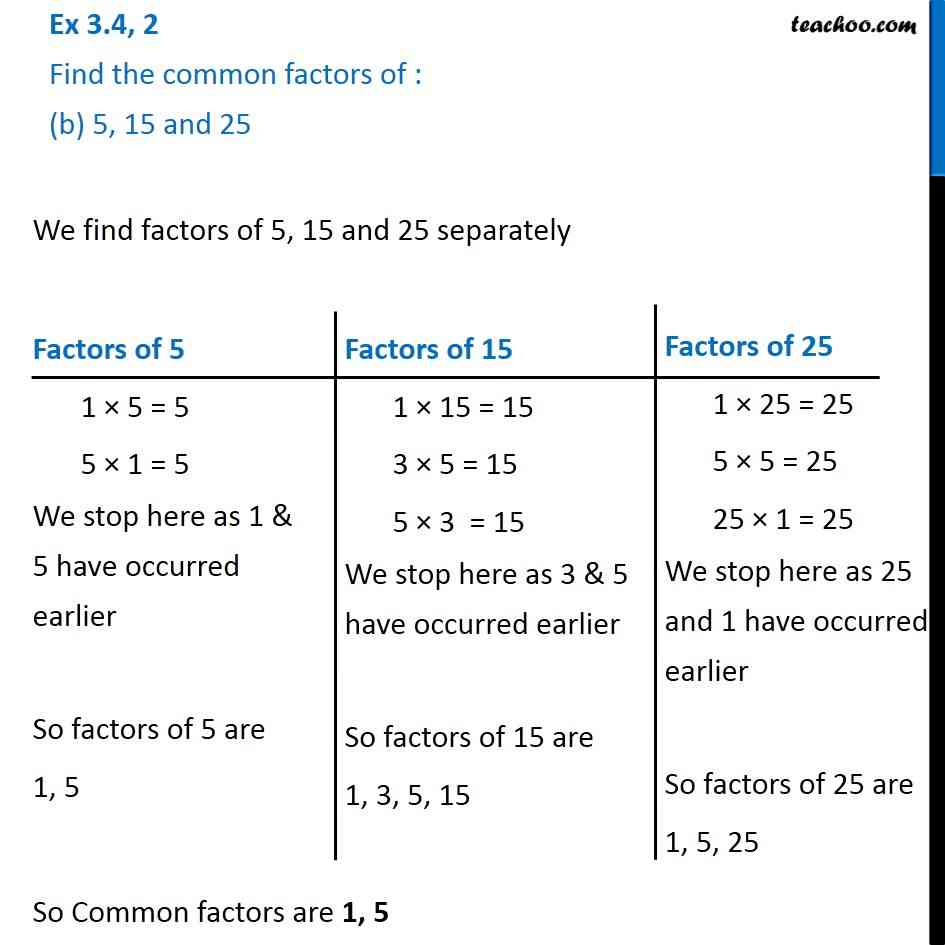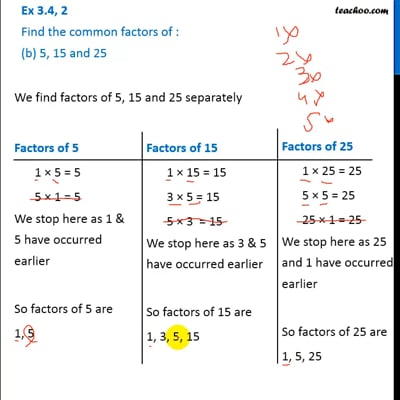Ex 3.4

Chapter 3 Class 6 Playing with Numbers
Serial order wiseThis video is only available for Teachoo black users

Introducing your new favourite teacher - Teachoo Black, at only ₹83 per month

### Transcript

Ex 3.4, 2 Find the common factors of : (b) 5, 15 and 25 We find factors of 5, 15 and 25 separately So Common factors are 1, 5 Factors of 5 1 × 5 = 5 5 × 1 = 5 We stop here as 1 & 5 have occurred earlier So factors of 5 are 1, 5 Factors of 15 1 × 15 = 15 3 × 5 = 15 5 × 3 = 15 We stop here as 3 & 5 have occurred earlier So factors of 15 are 1, 3, 5, 15 Factors of 25 1 × 25 = 25 5 × 5 = 25 25 × 1 = 25 We stop here as 25 and 1 have occurred earlier So factors of 25 are 1, 5, 25